# Vizing theorem

(diff) ← Older revision | Latest revision (diff) | Newer revision → (diff)

Letbe a graph (assumed to be finite, undirected and loop-less), and let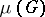be the maximum number of edges joining every pair of vertices. A simple graph will mean a graph without parallel edges. The chromatic index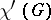is the least number of colours needed to colour the edges ofin such a way that no two adjacent edges are assigned the same colour (cf. also Graph colouring). If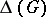is the maximum degree of, then, obviously,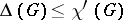. The origins of chromatic graph theory may be traced back to 1852, with the birth of the four-colour problem. The first paper on edge colourings appeared 1880, when P.G. Tait [a18] made the important observation that the four-colour conjecture is equivalent to the statement that every-regular planar graph has chromatic index. But after this, little was done. In 1916, D. König [a13] proved that ifis a bipartite graph (cf. also Graph, bipartite), then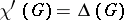. The first non-trivial upper bound has been obtained by C.E. Shannon [a17] in 1949, when he provedfor an arbitrary graph. The great breakthrough occurred in 1964, however, when V.G. Vizing [a19] gave the following surprisingly strong result, now known as Vizing's theorem: Ifis a graph, then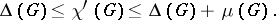In particular,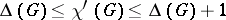ifis simple.

The cornerstone of Vizing's proof is a brilliant recolouring technique. Up to now (1999) all further proofs of his theorem are based more or less on this method (see, for example, [a5], [a10], and [a22]). In addition, the proof of Vizing's theorem can be used to obtain a polynomial-time algorithm to colour the edges of every graphwith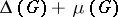colours. Nowadays, graphs are normally divided into two classes: those withare called class-1 graphs, and the others class-2 graphs. Class-2 graphs are relatively scarce, even among the simple graphs [a9]. In view of König's result, bipartite graphs are of class 1; however, the general question whether a given graph is class 1 or class 2 is very difficult, and it is known as the classification problem. This problem is extremely important and has received wide interest. The difficulty of the classification problem lies in the fact that it is-complete (cf. [a12]; Complexity theory), and that its solution would imply the four-colour theorem. Very little progress has been made for general graphs, and hence it is natural to consider this problem for special families. For regular graphs the so-called one-factorization conjecture goes back to the 1950s: Every simple-regular graph with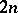vertices is class 1 (i.e.is-factorizable) when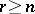. This conjecture is still open (1999). The best partial solutions go back to A.G. Chetwynd and A.J.W. Hilton [a7] and T. Niessen and L. Volkmann [a15]. They showed independently that the-factorization conjecture is valid if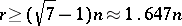. Appreciable progress has also been achieved in the particular case of planar graphs. On the one hand, if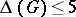, then a planar graphcan lie in either class 1 or class 2. On the other hand, Vizing [a20] has proved that every simple planar graphwith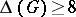is necessarily of class 1. However, the problem of determining what happens whenis eitherorremains open, and has led [a20] to the planar graph conjecture: Every simple planar graph of maximum degree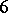oris of class 1.

The total chromatic numberis the least number of colours needed to colour the elements (edges and vertices) of a graphso that adjacent elements are coloured differently. The centre of interest lies in the still (1999) unproven total colouring conjecture of Vizing [a20] and M. Behzad [a3] that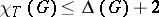for a simple graph. A.J.W. Hilton and H.R. Hind [a11] proved this conjecture by vertex colouring methods when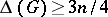, whereis the number of vertices of. Also, Vizing's theorem is a powerful tool in attacking this conjecture. For example, K.H. Chew [a6] has given a new proof of the Hilton–Hind result by transforming the total colouring problem to an edge-colouring problem. Using probabilistic methods, recently M. Molloy and B. Reed [a2] have obtained the following very deep theorem: There is an absolute constantsuch that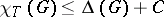for every simple graph. See [a23] for a thorough treatment of this topic.

In order to give reasonable upper bounds for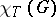, Vizing [a21] introduced the concept of list colouring: If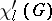is the list-chromatic index of a graph, then, obviously,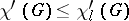. Vizing [a21] even posed the still open (1999) list colouring conjecture: Every graphsatisfies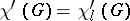. With a surprisingly short proof, F. Galvin [a1] showed that the list colouring conjecture is true for bipartite graphs. A.V. Kostochka [a14] proved that if all cycles in a simple graphare sufficiently long relative to, then. If the list colouring conjecture is true, then, according to Vizing's theorem, the same bound would hold for any simple graph.

The determination of the chromatic index can be transformed into a problem dealing with the chromatic number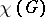. Namely, from the definition it is immediate that, whereis the line graph of the simple graph. If, in addition,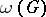is the clique number (cf. also Fixed-point property) of, then Vizing's theorem impliesTherefore, this bound is called [a16] the Vizing bound. L.W. Beineke [a4] characterized line graphs by nine forbidden induced subgraphs. Using only four forbidden induced subgraphs of Beineke's nine graphs, S.A. Choudom [a8] determined two superclasses of line graphs for which the Vizing bound is also valid. Thus, since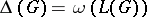ifis not a triangle, Choudom's result extends Vizing's theorem. The most general contribution in this area was found by H. Randerath [a16]: Ifis a simple graph containing neither a complete-graph with an edge missing nor a claw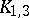with one subdivided edge as an induced subgraph, thenis equal toor.

How to Cite This Entry:
Vizing theorem. Encyclopedia of Mathematics. URL: http://encyclopediaofmath.org/index.php?title=Vizing_theorem&oldid=17012
This article was adapted from an original article by Lutz Volkmann (originator), which appeared in Encyclopedia of Mathematics - ISBN 1402006098. See original article